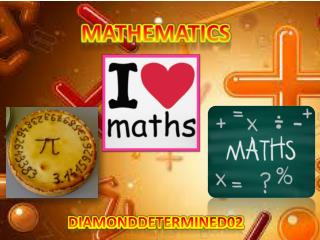DownloadDownload PresentationMathematics

# Mathematics

Download Presentation## Mathematics

- - - - - - - - - - - - - - - - - - - - - - - - - - - E N D - - - - - - - - - - - - - - - - - - - - - - - - - - -
##### Presentation Transcript

1. Mathematics DiamondDetermined02

2. Pi day is celebrated on the 14th of March. (Coming up soon!) This is because March is the third month of the year (3) and the 14th day, so 3.14... Pi approximation day is celebrated on the 22nd of July. This is because a fraction which is slightly higher than the actual value of pi, but is very close, is 22/7. July is the 7th month and it's celebrated on the 22nd. Pi Pi is an irrational number. It is the value of the ratio of the circumference of a circle to its diameter. It is approximately '3.14‘. 22/7 is the closest fraction to pi but there is no fraction which is equivalent exactly because pi has an infinite number of decimal places.

3. Algebra What is algebra? Algebra is a part of mathematics which letters and other symbols are used to represent numbers and quantities in formulae and equations. There are different types of algebra, such as: abstract algebra, linear algebra and universal algebra. When an equal sign (=) is used, the algebraic term is called an equation. A coefficient is a number which is followed by a letter or symbol in an algebraic expression.

4. Algebra (Continued) There is an equal sign, which means that this is an equation. In 4x, the coefficient is 4. This is because 4 comes before the letter x.

5. Pythagoras • The Pythagoras theorem is used to find the length of the hypotenuse. • Square the length of length and base of the triangle. • Add them up. • This will give you the square of the length of the hypotenuse. • Find the square root. • That is the length of the hypotenuse. Pythagoras was a Greek mathematician who lived approximately 2600 years ago. He is famous for finding a method to calculate the length of the largest side of a right-angled triangle. He started a group of mathematicians called the Pythagoreans, who worshipped numbers. They believed that the soul is immortal and that it goes through a cycle of re-births until it becomes pure.

6. Thank you for listening and Watching our reflection About mathematics 